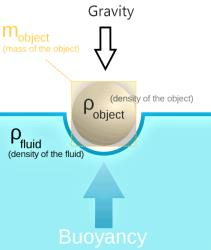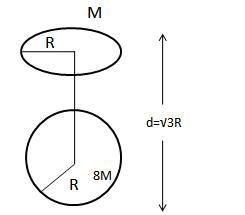Courses

# MCQ : Gravitation (Floatation) - 2

## 10 Questions MCQ Test Science Class 9 | MCQ : Gravitation (Floatation) - 2

Description
This mock test of MCQ : Gravitation (Floatation) - 2 for Class 9 helps you for every Class 9 entrance exam. This contains 10 Multiple Choice Questions for Class 9 MCQ : Gravitation (Floatation) - 2 (mcq) to study with solutions a complete question bank. The solved questions answers in this MCQ : Gravitation (Floatation) - 2 quiz give you a good mix of easy questions and tough questions. Class 9 students definitely take this MCQ : Gravitation (Floatation) - 2 exercise for a better result in the exam. You can find other MCQ : Gravitation (Floatation) - 2 extra questions, long questions & short questions for Class 9 on EduRev as well by searching above.
QUESTION: 1

Solution:
QUESTION: 2

Solution:
QUESTION: 3

### When a body floats in a liquid, its apparent weight is always

Solution:

Apparent weight is lessened by buoyancy, which occurs when an object is immersed in a fluid (a liquid or a gas). For example, an object immersed in water weighs less, according to a spring balance, than the same object in air. The apparent weight of a floating object is zero.

QUESTION: 4

A mass weighing W can float if the upthrust U is related as

Solution:

If the weight of water is greater than the upward buoyant force acting on it, then net force W−U acts downwards. Hence the body displaces downwards, that is, sink.

QUESTION: 5

The relative density of a solid is 0.6. It floats in water with

Solution:
QUESTION: 6

The least value of apparent weight of a body in a fluid is

Solution:
QUESTION: 7

The pressure exerted by man on earth is minimum when he

Solution:
QUESTION: 8

An empty closed drum and a filled drum of same dimension will bring

Solution:

Explanation : An empty closed drum and a filled drum of same dimension will bring same upthrust and same volume

QUESTION: 9

The relation between force F, pressure P and area A is

Solution:
QUESTION: 10

A girl stands on a box having 60 cm length, 40 cm breadth and 20 cm width in three ways.In which of the following cases, pressure exerted by the brick will be

Solution:

Track your progress, build streaks, highlight & save important lessons and more!

### Similar Content### Related tests